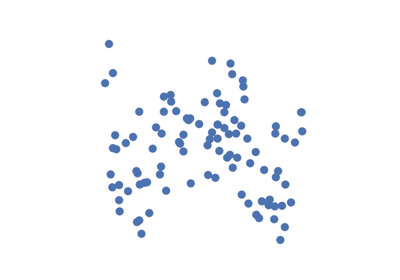# w_shaped¶

hyppo.tools.w_shaped(n, p, noise=False, low=- 1, high=1)

W-Shaped simulation.

W-Shaped $$(X, Y) \in \mathbb{R}^p \times \mathbb{R}$$: $$\mathcal{U}(-1, 1)^p$$,

$\begin{split}X &\sim \mathcal{U}(-1, 1)^p \\ Y &= \left[ \left( (w^T X)^2 - \frac{1}{2} \right)^2 + \frac{w^T U}{500} \right] + 0.5 \kappa \epsilon\end{split}$
Parameters
Returns

x,y (ndarray) -- Simulated data matrices. x and y have shapes (n, p) and (n, 1) where n is the number of samples and p is the number of dimensions.

## Examples using hyppo.tools.w_shaped`¶